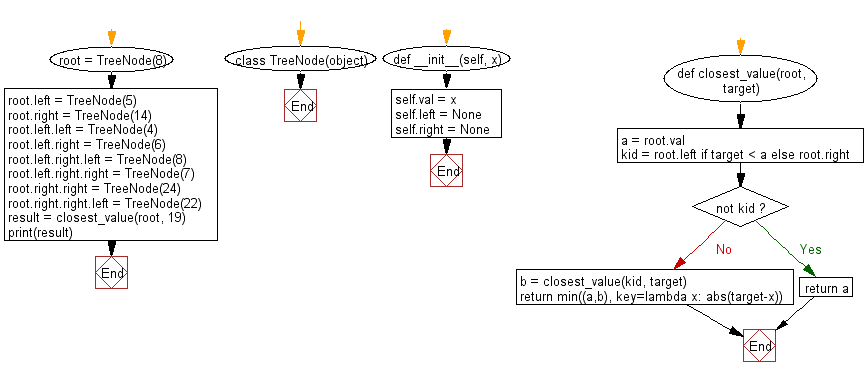﻿ Python Binary Search Tree: Find the closest value of a target in a given non-empty Binary Search Tree (BST) of unique values - w3resource

# Python Binary Search Tree: Find the closest value of a target in a given non-empty Binary Search Tree (BST) of unique values

## Python Binary Search Tree: Exercise-2 with Solution

Write a Python program to find the closest value to a given target value in a given non-empty Binary Search Tree (BST) of unique values.

Sample Solution:

Python Code:

``````class TreeNode(object):
def __init__(self, x):
self.val = x
self.left = None
self.right = None

def closest_value(root, target):
a = root.val
kid = root.left if target < a else root.right
if not kid:
return a
b = closest_value(kid, target)
return min((a,b), key=lambda x: abs(target-x))

root = TreeNode(8)
root.left = TreeNode(5)
root.right = TreeNode(14)
root.left.left = TreeNode(4)
root.left.right = TreeNode(6)
root.left.right.left = TreeNode(8)
root.left.right.right = TreeNode(7)
root.right.right = TreeNode(24)
root.right.right.left = TreeNode(22)

result = closest_value(root, 19)
print(result)
```
```

Sample Output:

```22
```

Flowchart:Python Code Editor:

What is the difficulty level of this exercise?

Test your Programming skills with w3resource's quiz.

﻿

## Python: Tips of the Day

Joining Collection:

```name = ["Owen", "Eddie"]
print(" ".join(name))
```
`Owen Eddie`

We are closing our Disqus commenting system for some maintenanace issues. You may write to us at reach[at]yahoo[dot]com or visit us at Facebook